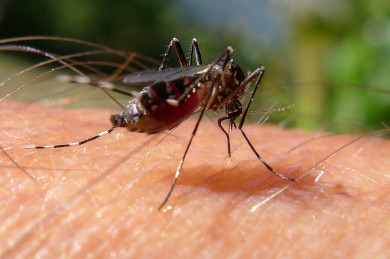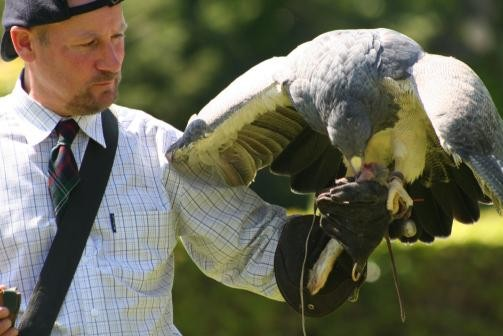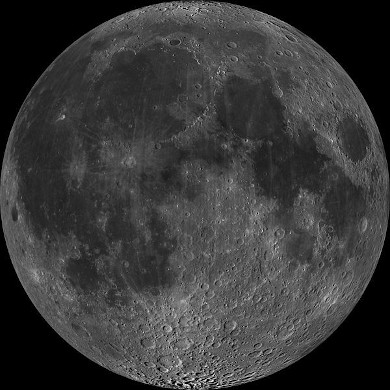Home Practice
For learners and parents For teachers and schools
Textbooks
Full catalogue
Pricing SupportLog in

We think you are located in United States. Is this correct?

# Test yourself now

High marks in science are the key to your success and future plans. Test yourself and learn more on Siyavula Practice.

# Chapter 2: Momentum and impulse

## 2.1 Introduction (ESCJ6)

In Grade 10 we studied motion but not what caused the motion, in Grade 11 we learnt about forces and how they can alter the motion of an object. In this chapter we will focus on what happens when two bodies undergo a contact interaction and how their motion is affected. We learn more about how force and motion are related. We are introduced to two new concepts, momentum and impulse.

We can begin by considering some scenarios to set the context. Most people have some intuition for physics based on their everyday experiences but they haven't formalised it. We can use our intuitive answers to lead into more structured thinking about physical events.

Momentum transfer doesn't require a contact interaction but we won't consider any non-contact scenarios in this chapter.

Everyone has experienced a mosquito landing on their arm and it can happen quite unnoticed. Consider the case of a falcon landing on your arm (ignore the sharp claws for now). You would definitely notice, why? What makes a mosquito different to a falcon? Would you still notice if the mosquito flew the same way as a falcon, or if the falcon copied the flight of a mosquito before landing? You probably would still notice, but try to think about what makes them so different.Look at a motorcycle, motorcar and truck. Which of them is more likely to result in less damage in a collision situation, why? What factors would you change to reduce potential damage.Why is the Moon's orbit largely unaffected when it is hit by asteroid?The factors that come up in these considerations are how fast things are moving and how massive they are. A falcon moving at the same speed as a mosquito still has a much larger mass. Even if a mosquito moved as fast as a falcon it wouldn't bother us because the mass of a mosquito is so small.

If a motorcycle, motorcar and truck were all moving at the same speed then it would be much safer to be in a collision with the motorcycle but a truck doesn't have to be moving as fast as a motorcycle to have a huge impact in a collision because of its large mass.

There is an interplay between mass and speed (velocity to be precise) that governs what would happen if these objects came into contact with another object. There are two quantities that depend on mass and velocity, kinetic energy and momentum. Kinetic energy is something that we learnt about previous. Momentum is different to kinetic energy and is what we will focus on in the first part of this chapter. In the second part we will cover some of the differences between kinetic energy and momentum.

• Units and unit conversions - Physical Sciences, Grade 10, Science skills
• Equations - Mathematics, Grade 10, Equations and inequalities
• Techniques of vector addition - Physical Sciences, Grade 10, Vectors and scalars
• Newton's laws - Physical Sciences, Grade 11, Forces

The following must be covered by the end of this chapter:

Momentum:

• The definition of momentum.
• The calculations done on momentum using p = mv and the change in momentum under the influence of a resultant force.
• The understanding of the vector nature of momentum and the applications concerning momentum.
• Representing momentum using vector diagrams.

Newton's second law expressed in terms of momentum:

• State Newton's second law in terms of momentum.
• Expression of Newton's second law in terms of symbols.
• Emphasis on the vector nature of momentum
• The relationship between the net force and change in momentum for a variety of motions by using different examples.
• Do calculations on the change in momentum when a resultant force acts on an object to change the velocity.

Conservation of momentum:

• Definition of :
• A system
• Internal forces
• External forces
• Isolated system
• State the law of conservation of momentum.
• Explain the difference between elastic and inelastic collisions by using the concept of kinetic energy before and after a collision.
• Do calculations on the principle of conservation of momentum.
• Do practical demonstrations on the principle of conservation of momentum.
• Applications of the principle of conservation of momentum.

Impulse:

• Definition of impulse.
• The understanding of the vector nature of impulse.
• The change in momentum and impulse are equal to each other (impulse-momentum theorem).
• Calculations done by using the impulse-momentum theorem.
• Units and unit conversions - Physical Sciences, Grade 10, Science skills
• Equations - Mathematics, Grade 10, Equations and inequalities
• Techniques of vector addition - Physical Sciences, Grade 10, Vectors and scalars
• Newton's laws - Physical Sciences, Grade 11, Forces